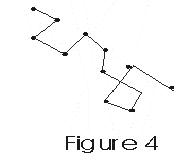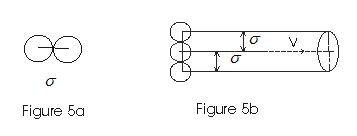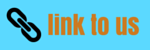# Kinetic Theory Of Gases

## 10. Mean free Path

• On the basis of kinetic theory of gases, it is assumed that the molecules of a gas are continously collilding against each other.
• Molecules move in straight line with constant speeds between two successive collisions.
• Thus path of a single molecule is a series of zig-zag paths of different lengths as shown in fig -.• These paths of different lengths are called free paths of the molecule

• Mean Free Path is the averege distance traversed by molecule between two successive collisions.
• If s is the Total path travelled in Ncoll coilisions, then mean free path
λ= s/Ncoll
Expression for mean free path :
• Consider a gas containing n molecules per unit volume.
• We assume that only one molecule which is under consideration is in motion while all others are at rest.
• If σ is the diameter of each molecule then the moving molecule will collide with all these molecules where centers lie within a distance from its centre as shown in fig

<• If v is the velocity of the moving molecule then in one second it will collide with all moleculeswith in a distance σ between the centres.
• In one second it sweeps a volume πσ2v where any other molecule will collide with it.
• If n is the total number of molecules per unit volume, then nπσ2v is number of collisions a molecule suffers in one second.
• If v is the distance traversed by molecule in one second then mean free path is given by
λ = total distance traversed in one second /no. of collision suffered by the molecules
=v/πσ2vn
=1/πσ2n

• This expression was derived with the assumption that all the molecules are at rest except the one which is colliding with the others.
• However this assumption does not represent actual state of affiar.
• More exact statement can be derived considering that all molecules are moving with all possible velocities in all possible directions.
• More exact relation found using distribution law of molecular speeds is
λ=1/(√2)πσ2n
its derivation is beyond our scope.